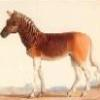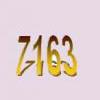# Search by Topic

Filter by: Content type:
Age range:
Challenge level:

### There are 21 results

Broad Topics > Numbers and the Number System > Prime factors### Fac-finding

##### Age 14 to 16 Challenge Level:

Lyndon chose this as one of his favourite problems. It is accessible but needs some careful analysis of what is included and what is not. A systematic approach is really helpful.### One to Eight

##### Age 11 to 14 Challenge Level:

Complete the following expressions so that each one gives a four digit number as the product of two two digit numbers and uses the digits 1 to 8 once and only once.### Why 24?

##### Age 14 to 16 Challenge Level:

Take any prime number greater than 3 , square it and subtract one. Working on the building blocks will help you to explain what is special about your results.### Em'power'ed

##### Age 16 to 18 Challenge Level:

Find the smallest numbers a, b, and c such that: a^2 = 2b^3 = 3c^5 What can you say about other solutions to this problem?### Flow Chart

##### Age 11 to 14 Challenge Level:

The flow chart requires two numbers, M and N. Select several values for M and try to establish what the flow chart does.### Rarity

##### Age 16 to 18 Challenge Level:

Show that it is rare for a ratio of ratios to be rational.### There's Always One Isn't There

##### Age 14 to 16 Challenge Level:

Take any pair of numbers, say 9 and 14. Take the larger number, fourteen, and count up in 14s. Then divide each of those values by the 9, and look at the remainders.### Different by One

##### Age 14 to 16 Challenge Level:

Make a line of green and a line of yellow rods so that the lines differ in length by one (a white rod)### Bubble Trouble

##### Age 5 to 7 Challenge Level:

Can you find just the right bubbles to hold your number?### Can it Be

##### Age 16 to 18 Challenge Level:

When if ever do you get the right answer if you add two fractions by adding the numerators and adding the denominators?### Public Key Cryptography

##### Age 16 to 18

An introduction to the ideas of public key cryptography using small numbers to explain the process. In practice the numbers used are too large to factorise in a reasonable time.### Factorial Fun

##### Age 16 to 18 Challenge Level:

How many divisors does factorial n (n!) have?### Just Repeat

##### Age 11 to 14 Challenge Level:

Think of any three-digit number. Repeat the digits. The 6-digit number that you end up with is divisible by 91. Is this a coincidence?### Powerful Factorial

##### Age 11 to 14 Challenge Level:

6! = 6 x 5 x 4 x 3 x 2 x 1. The highest power of 2 that divides exactly into 6! is 4 since (6!) / (2^4 ) = 45. What is the highest power of two that divides exactly into 100!?### Great Granddad

##### Age 11 to 14 Challenge Level:

Great Granddad is very proud of his telegram from the Queen congratulating him on his hundredth birthday and he has friends who are even older than he is... When was he born?### Factoring a Million

##### Age 14 to 16 Challenge Level:

In how many ways can the number 1 000 000 be expressed as the product of three positive integers?### Factoring Factorials

##### Age 11 to 14 Challenge Level:

Find the highest power of 11 that will divide into 1000! exactly.### Thirty Six Exactly

##### Age 11 to 14 Challenge Level:

The number 12 = 2^2 × 3 has 6 factors. What is the smallest natural number with exactly 36 factors?### Gaxinta

##### Age 11 to 14 Challenge Level:

A number N is divisible by 10, 90, 98 and 882 but it is NOT divisible by 50 or 270 or 686 or 1764. It is also known that N is a factor of 9261000. What is N?### A Biggy

##### Age 14 to 16 Challenge Level:

Find the smallest positive integer N such that N/2 is a perfect cube, N/3 is a perfect fifth power and N/5 is a perfect seventh power.### Some Cubes

##### Age 16 to 18 Challenge Level:

The sum of the cubes of two numbers is 7163. What are these numbers?